# 跟我学Python图像处理丨带你入门OpenGL

2022-12-09 16:00 https://my.oschina.net/u/4526289/blog/5606624 华为云开发者联盟 次阅读 条评论

## 一.OpenGL入门知识

### 1.什么是OpenGL

OpenGL可用于设置所需的对象、图像和操作，以便开发交互式的3维计算机图形应用程序。OpenGL被设计为一个现代化的、硬件无关的接口，因此我们可以在不考虑计算机操作系统或窗口系统的前提下，在多种不同的图形硬件系统上，或者完全通过软件的方式实现OpenGL的接口。OpenGL的高效实现（利用了图形加速硬件）存在于Windows，部分UNIX平台和Mac OS。这些实现一般由显示设备厂商提供，而且非常依赖于该厂商提供的硬件。OpenGL规范由1992年成立的OpenGL架构评审委员会（ARB）维护。ARB由一些对创建一个统一的、普遍可用的API特别感兴趣的公司组成。到了今天已经发布了非常多的OpenGL版本，以及大量构建于OpenGL之上以简化应用程序开发过程的软件库。这些软件库大量用于视频游戏、科学可视化和医学软件的开发，或者只是用来显示图像。

• 从OpenGL的几何图元中设置数据，用于构建形状
• 将输入图元的数学描述转换为与屏幕位置对应的像素片元（fragment），这一步也称作光栅化（rasterization）
• 如果有必要，还需要对每个片元执行一些额外的操作，例如判断片元对应的对象是否可见，或者将片元的颜色与当前屏幕位置的颜色进行融合

### 2.OpenGL安装

``````cd C:\Software\Program Software\Python37\Scripts
pip install pyopengl````````````pip install D:\PyOpenGL-3.1.5-cp37-cp37m-win_amd64.whl
pip install D:\PyOpenGL-3.1.5-cp37-cp37m-win32.whl``````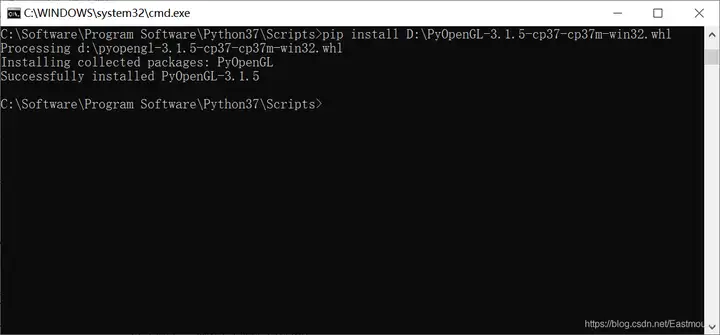## 二.OpenGL入门程序

### 1.OpenGL绘制正方形

``````# -*- coding: utf-8 -*-
from OpenGL.GL import *
from OpenGL.GLU import *
from OpenGL.GLUT import *
# 绘制图像函数
def display():
# 清除屏幕及深度缓存
glClear(GL_COLOR_BUFFER_BIT|GL_DEPTH_BUFFER_BIT)
# 设置红色
glColor3f(1.0, 0.0, 0.0)
# 开始绘制四边形
# 绘制四个顶点
glVertex3f(-0.5, -0.5, 0.0)
glVertex3f(0.5, -0.5, 0.0)
glVertex3f(0.5, 0.5, 0.0)
glVertex3f(-0.5, 0.5, 0.0)
# 结束绘制四边形
glEnd()
# 清空缓冲区并将指令送往硬件执行
glFlush()
# 主函数
if __name__ == "__main__":
# 使用glut库初始化OpenGL
glutInit()
# 显示模式 GLUT_SINGLE无缓冲直接显示|GLUT_RGBA采用RGB(A非alpha)
glutInitDisplayMode(GLUT_SINGLE | GLUT_RGBA)
# 设置窗口位置大小
glutInitWindowSize(400, 400)
# 创建窗口
glutCreateWindow("eastmount")
# 调用display()函数绘制图像
glutDisplayFunc(display)
# 进入glut主循环
glutMainLoop()``````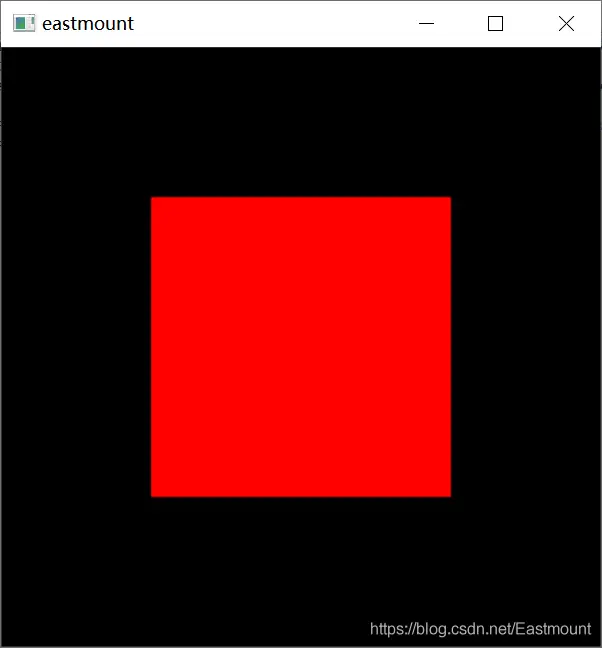• 主函数使用glut库初始化OpenGL
glutInit()
• 设置显示模式并初始化glut窗口（画布）
glutInitDisplayMode(GLUT_SINGLE | GLUT_RGBA)
glutInitWindowSize(400, 400)
glutCreateWindow(“eastmount”)
• 注册绘制图像的回调函数，如display()
glutDisplayFunc(display)
• 绘制图像display函数，包括清除画布、设置颜色、绘制图元、设置定点、结束绘制、刷新执行
glClear(GL_COLOR_BUFFER_BIT|GL_DEPTH_BUFFER_BIT)
glColor3f(1.0, 0.0, 0.0)
glVertex3f(-0.5, -0.5, 0.0)
glVertex3f(0.5, -0.5, 0.0)
glVertex3f(0.5, 0.5, 0.0)
glVertex3f(-0.5, 0.5, 0.0)
glEnd()
glFlush()
• 进入glut主循环
glutMainLoop()

### 2.OpenGL绘制水壶

``````# -*- coding: utf-8 -*-
from OpenGL.GL import *
from OpenGL.GLU import *
from OpenGL.GLUT import *
# 绘制图像函数
def drawFunc():
# 清除屏幕及深度缓存
glClear(GL_COLOR_BUFFER_BIT|GL_DEPTH_BUFFER_BIT)
# 设置绕轴旋转(角度,x,y,z)
glRotatef(0.1, 5, 5, 0)
# 绘制实心茶壶
# glutSolidTeapot(0.5)
# 绘制线框茶壶
glutWireTeapot(0.5)
# 刷新显示图像
glFlush()
# 主函数
if __name__ == "__main__":
# 使用glut库初始化OpenGL
glutInit()
# 显示模式 GLUT_SINGLE无缓冲直接显示|GLUT_RGBA采用RGB(A非alpha)
glutInitDisplayMode(GLUT_SINGLE | GLUT_RGBA)
# 设置窗口位置及大小
glutInitWindowPosition(0, 0)
glutInitWindowSize(400, 400)
# 创建窗口
glutCreateWindow("CSDN Eastmount")
# 调用display()函数绘制图像
glutDisplayFunc(drawFunc)
# 设置全局的回调函数
# 当没有窗口事件到达时,GLUT程序功能可以执行后台处理任务或连续动画
glutIdleFunc(drawFunc)
# 进入glut主循环
glutMainLoop()``````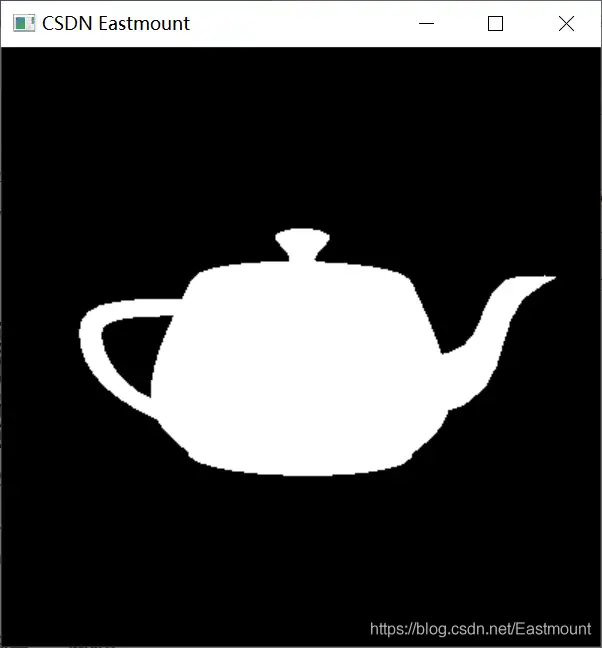### 3.OpenGL绘制多个图形

``````# -*- coding: utf-8 -*-
from OpenGL.GL import *
from OpenGL.GLU import *
from OpenGL.GLUT import *
# 绘制图像函数
def display():
# 清除屏幕及深度缓存
glClear(GL_COLOR_BUFFER_BIT|GL_DEPTH_BUFFER_BIT)
# 绘制线段
glBegin(GL_LINES)
glVertex2f(-1.0, 0.0) # 左下角顶点
glVertex2f(1.0, 0.0) # 右下角顶点
glVertex2f(0.0, 1.0) # 右上角顶点
glVertex2f(0.0, -1.0) # 左上角顶点
glEnd()
# 绘制顶点
glPointSize(10.0)
glBegin(GL_POINTS)
glColor3f(1.0, 0.0, 0.0) # 红色
glVertex2f(0.3, 0.3)
glColor3f(0.0, 1.0, 0.0) # 绿色
glVertex2f(0.5, 0.6)
glColor3f(0.0, 0.0, 1.0) # 蓝色
glVertex2f(0.9, 0.9)
glEnd()
# 绘制四边形
glColor3f(1.0, 1.0, 0)
glVertex2f(-0.2, 0.2)
glVertex2f(-0.2, 0.5)
glVertex2f(-0.5, 0.5)
glVertex2f(-0.5, 0.2)
glEnd()
# 绘制多边形
glColor3f(0.0, 1.0, 1.0)
glPolygonMode(GL_FRONT, GL_LINE)
glPolygonMode(GL_BACK, GL_FILL)
glBegin(GL_POLYGON)
glVertex2f(-0.5, -0.1)
glVertex2f(-0.8, -0.3)
glVertex2f(-0.8, -0.6)
glVertex2f(-0.5, -0.8)
glVertex2f(-0.2, -0.6)
glVertex2f(-0.2, -0.3)
glEnd()
# 绘制三角形
glColor3f(1.0, 1.0, 1.0)
glPolygonMode(GL_FRONT, GL_FILL)
glPolygonMode(GL_BACK, GL_LINE)
glBegin(GL_TRIANGLES)
glVertex2f(0.5, -0.5)
glVertex2f(0.3, -0.3)
glVertex2f(0.2, -0.6)
# 结束绘制四边形
glEnd()
# 清空缓冲区并将指令送往硬件执行
glFlush()
# 主函数
if __name__ == "__main__":
# 使用glut库初始化OpenGL
glutInit()
# 显示模式 GLUT_SINGLE无缓冲直接显示|GLUT_RGBA采用RGB(A非alpha)
glutInitDisplayMode(GLUT_SINGLE | GLUT_RGBA)
# 设置窗口位置及大小
glutInitWindowSize(400, 400)
glutInitWindowPosition(500, 300)
# 创建窗口
glutCreateWindow("CSDN Eastmount")
# 调用display()函数绘制图像
glutDisplayFunc(display)
# 进入glut主循环
glutMainLoop()``````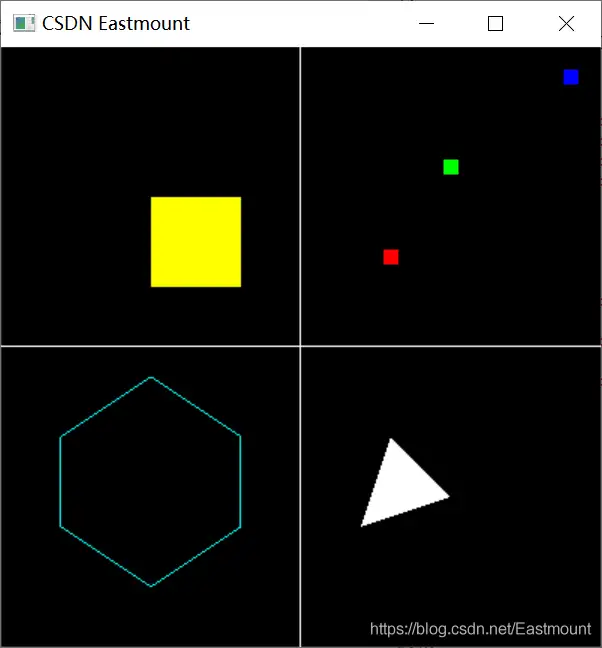### 4.OpenGL绘图代码及原理详解

(1) 核心函数

• glutInit()：对GLUT进行初始化，该函数必须在其它的GLUT使用之前调用一次。其格式比较死板，一般glutInit()直接调用即可。
• glutInitWindowPosition()：设置窗口在屏幕中的位置。
• glutInitWindowSize()：设置窗口的大小，两个参数表示长度和宽度。
• glutCreateWindow()：根据当前设置的信息创建窗口，参数将作为窗口的标题。需要注意的是，当窗口被创建后，并不是立即显示到屏幕上，需要调用glutMainLoop()才能看到窗口。
• glutDisplayFunc()：设置一个函数，当需要进行画图时，这个函数就会被调用，通常用来调用绘制图形函数。
• glutMainLoop()：进行一个消息循环，大家需要知道这个函数可以显示窗口，并且等待窗口关闭后才会返回。

• glClear()：清除，其中参数GL_COLOR_BUFFER_BIT表示清除颜色，GL_DEPTH_BUFFER_BIT表示清除深度。
• glRectf()：画一个矩形，四个参数分别表示位于对角线上的两个点的横、纵坐标。
• glFlush()：刷新显示图像，保证前面的OpenGL命令立即执行，而不是让它们在缓冲区中等待。
• OpenGL要求指定顶点的命令（glVertex2f）必须包含在glBegin()函数和glEnd()函数之间执行。

(2) 绘制顶点

• glVertex2d
• glVertex2f
• glVertex3f
• glVertex3fv

• glVertex2i(1, 3)
• glVertex2f(1.0, 3.0)
• glVertex3f(1.0, 3.0, 1.0)
• glVertex4f(1.0, 3.0, 0.0, 1.0)

(3) 设置颜色

• glColor3f(1.0，0.0，0.0) #红色
• glColor3f(0.0，1.0，0.0) #绿色
• glColor3f(0.0，0.0，1.0) #蓝色
• glColor3f(1.0，1.0，1.0) #白色
• glColor4f(0.0，1.0，0.0，0.0) #红色且不透明度
• glColor3ub(255, 0, 0) #红色

(4) 绘制基本图形

• GL_POINTS：绘制顶点
• GL_LINES：绘制线段
• GL_LINE_STRIP：绘制连续线段
• GL_LINE_LOOP：绘制闭合的线段
• GL_POLYGON：绘制多边形
• GL_TRIANGLES：绘制三角形
• GL_TRIANGLE_STRIP：绘制连续三角形
• GL_TRIANGLE_FAN：绘制多个三角形组成的扇形## 三.OpenGL基础知识

### 1.OpenGL语法

OpenGL程序的基本结构通常包括——初始化物体渲染所对应的状态、设置需要渲染的物体。渲染（render）表示计算机从模型创建最终图像的过程，OpenGL只是其中一种渲染系统。模型（model）或者场景对象是通过几何图元，比如点、线和三角形来构建的，而图元与模型的顶点（vertex）也存在着各种对应的关系。### 2.老式OpenGL vs 现代OpenGL

(1) 老式OpenGL(2) 现代OpenGL• 三维几何图形定义（VBO等）。 在第一步，通过定义在三维空间中的三角形的顶点，并指定每个顶点相关联的颜色，我们定义了三维几何图形。
• 顶点着色器。 接下来，变换这些顶点：第一次变换将这些顶点放在三维空间中，第二次变换将三维坐标投影到二维空间。根据照明等因素，对应顶点的颜色值也在这一步中计算，这在代码中通常称为“顶点着色器”。
• 光栅化。 将几何图形“光栅化”（从几何物体转换为像素）。
• 片段着色器。 针对每个像素，执行另一个名为“片段着色器”的代码块。正如顶点着色器作用于三维顶点，片段着色器作用于光栅化后的二维像素。
• 帧缓冲区操作（深度测试、混合等）。 最后，像素经过一系列帧缓冲区操作，其中，它经过“深度缓冲区检验”（检查一个片段是否遮挡另一个）、“混合”（用透明度混合两个片段）以及其他操作，其当前的颜色与帧缓冲区中该位置已有的颜色结合。
• 帧缓冲区。 这些变化最终体现在最后的帧缓冲区上，通常显示在屏幕上。

PS：该部分参考Mahesh Venkitachalam大神编写的《Python极客项目编程》，代码可以查看：https://github.com/electronut/pp

### 3.OpenGL绘制时钟

``````# -*- coding: utf-8 -*-
from OpenGL.GL import *
from OpenGL.GLU import *
from OpenGL.GLUT import *
import math
import time
h = 0
m = 0
s = 0
# 绘制图像函数
def Draw():
PI = 3.1415926
R = 0.5
TR = R - 0.05
glClear(GL_COLOR_BUFFER_BIT)
glLineWidth(5)
glBegin(GL_LINE_LOOP)
for i in range(100):
glVertex2f(R * math.cos(2 * PI / 100 * i), R * math.sin(2 * PI / 100 * i))
glEnd()
glLineWidth(2)
for i in range(100):
glBegin(GL_LINES)
glVertex2f(TR * math.sin(2 * PI / 12 * i), TR * math.cos(2 * PI / 12 * i))
glVertex2f(R * math.sin(2 * PI / 12 * i), R * math.cos(2 * PI / 12 * i))
glEnd()
glLineWidth(1)
h_Length = 0.2
m_Length = 0.3
s_Length = 0.4
count = 60.0
s_Angle = s / count
count *= 60
m_Angle = (m * 60 + s) / count
count *= 12
h_Angle = (h * 60 * 60 + m * 60 + s) / count
glLineWidth(1)
glBegin(GL_LINES)
glVertex2f(0.0, 0.0)
glVertex2f(s_Length * math.sin(2 * PI * s_Angle), s_Length * math.cos(2 * PI * s_Angle))
glEnd()
glLineWidth(5)
glBegin(GL_LINES)
glVertex2f(0.0, 0.0)
glVertex2f(h_Length * math.sin(2 * PI * h_Angle), h_Length * math.cos(2 * PI * h_Angle))
glEnd()
glLineWidth(3)
glBegin(GL_LINES)
glVertex2f(0.0, 0.0)
glVertex2f(m_Length * math.sin(2 * PI * m_Angle), m_Length * math.cos(2 * PI * m_Angle))
glEnd()
glLineWidth(1)
glBegin(GL_POLYGON)
for i in range(100):
glVertex2f(0.03 * math.cos(2 * PI / 100 * i), 0.03 * math.sin(2 * PI / 100 * i));
glEnd()
glFlush()
# 更新时间函数
def Update():
global h, m, s
t = time.localtime(time.time())
h = int(time.strftime('%H', t))
m = int(time.strftime('%M', t))
s = int(time.strftime('%S', t))
glutPostRedisplay()
# 主函数
if __name__ == "__main__":
glutInit()
glutInitDisplayMode(GLUT_SINGLE | GLUT_RGBA)
glutInitWindowSize(400, 400)
glutCreateWindow("My clock")
glutDisplayFunc(Draw)
glutIdleFunc(Update)
glutMainLoop() ``````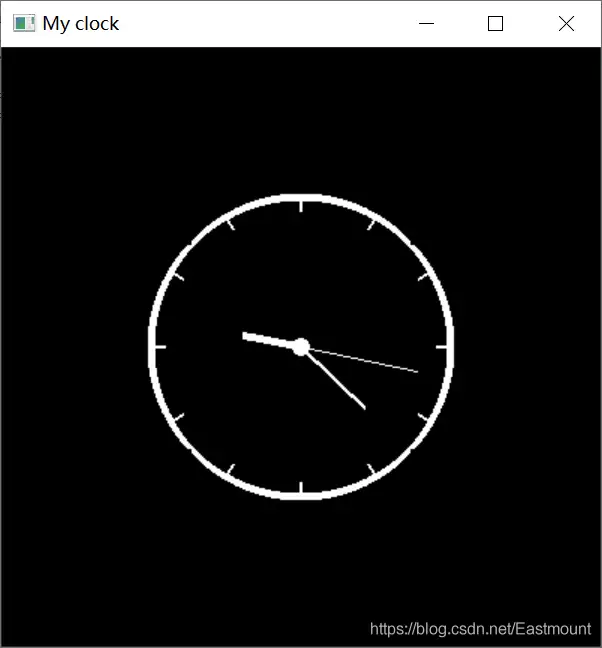## 参考文献：

 《OpenGL编程指南（第8版）》 作者：Dave Shreiner Granham Sellers等，王锐 译
 《Python极客项目编程》 作者：Mahesh Venkitachalam，王海鹏 译
 《OpenGL编程精粹》杨柏林 陈根浪 徐静 编著
 写给 python 程序员的 OpenGL 教程 - 许老师(天元浪子)
 Python之OpenGL笔记(2)：现代OpenGL编程常用的几个通用函数 - 大龙老师
 python3+OpenGL环境配置 - GraceSkyer老师
 VS2012下基于Glut OpenGL显示一些立体图形示例程序 - yearafteryear老师
 Python——OpenGL - 白季飞龙老师
 python+opengl显示三维模型小程序 - xiaoge2016
 https://github.com/electronut/pp

 0人感动 0人路过 0人高兴 0人难过 0人搞笑 0人无聊 0人愤怒 0人同情OpenHarmony是开放原子开源基金会（OpenAtom Foundation）旗下开源... [详细]